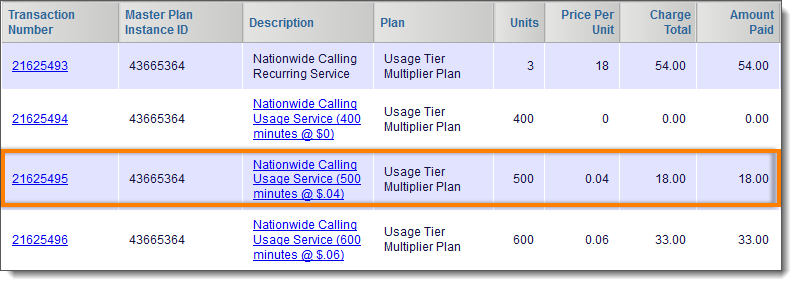# Usage Tier Multiplier Example

## Overview

Outlined below is an example of how usage is rated for a service that has Usage Tier Multiplier enabled. In this scenario, 3 instances of the service's master plan are purchased.

Note: Usage Tier Multiplier can be enabled for all pricing methods and applies whether real-time usage rating is enabled or not. In the below example, the service has a standard tier pricing method and real-time usage rating is turned on.

## Calculating Usage Rating with Usage Tier Multiplier

To understand the usage rating in this scenario, it is important to consider the following components:

• The adjusted rate schedule from Usage Tier Multiplier being enabled
• The usage rating, calculated by the adjusted rate schedule
• The factored unit rate that appears on the invoice

Because a customer purchased 3 instances of the master plan, and Usage Tier Multiplier is enabled for the service, the rate schedule is adjusted so that the range of units in each tier is multiplied by 3. The table below shows the service's rate schedule (on the left) and the rate schedule adjustment due to 3 instances of the master plan being purchased (on the right).

Rate Schedule Usage Tier Multiplier Rate Schedule
Units Rate Units Rate

1 – 200

\$0.00

1 – 600

\$0.00

201 – 400

\$0.06

601 – 1200

\$0.06

401 –600

\$0.05

1201 – 1800

\$0.05

601 – Infinity

\$0.03

1801 – Infinity

\$0.03

### Usage Rating Calculation

1,500 units of usage are loaded in 3 different intervals over a billing period, as shown in the table below. Because the total charge on the invoice for a usage-based service is the sum of the charges for each usage load, the total invoice charge for this service is \$51 (\$0 + \$18 + \$33).

Units Usage Rating Total Charge Factored Unit Rate Calculation Explanation

400 units

400 units x \$0.00 = \$0

\$0

The first usage load of 400 units is rated at \$0.00 and has no charge since it falls into tier 1 of the Usage Tier Multiplier.

500 units

200 units x \$0.00 = \$0

300 units x \$0.06 = \$18

\$18

\$18 / 500 units = \$.036

(rounded to \$.04 on invoice)

The second usage load of 500 falls over two tiers. The first 200 units falls into tier 1 of the Usage Tier Multiplier and have no charge. The remaining 300 units fall into tier 2 of the Usage Tier Multiplier and are charged at .06 per unit for a total of \$18.

600 units

300 units x \$0.06 = \$18

300 units x \$0.05 = \$15

\$33

\$33 / 600 units = \$.055

(rounded to \$.06 on invoice)

The third usage load of 600 units again falls over two tiers. The first 300 units fall into tier 2 of the Usage Tier Multiplier and are charged at .06 per unit for a total of \$18. The remaining 300 units fall into tier 3 of the Usage Tier Multiplier and are charged at .05 per unit for a total of \$15.

### Factored Unit Rate

To understand how the invoice calculates the total charge for the service, it is important to realize how the factored unit rate is calculated for each usage load charge. A factored rate is the adjusted rate per unit for a usage load that falls across tiers of a rate schedule. You can use the below formula to get the factored rate per unit.

Factored Rate Per Unit = Total Charge of Usage Load / Number of Units in the Usage Load

For instance, in the second usage load of the above scenario, 500 units were loaded for a total charge of \$18 (200 units at \$0.00 and 300 units at \$0.06). This means the factored rate for each unit in this usage load is \$18 / 500 units, which is equal to \$0.036 (rounded to \$0.04 on the invoice).

The factored unit can be seen as the Price Per Unit on the generated invoice (Account > Statements & Invoices > Invoices), as shown below.